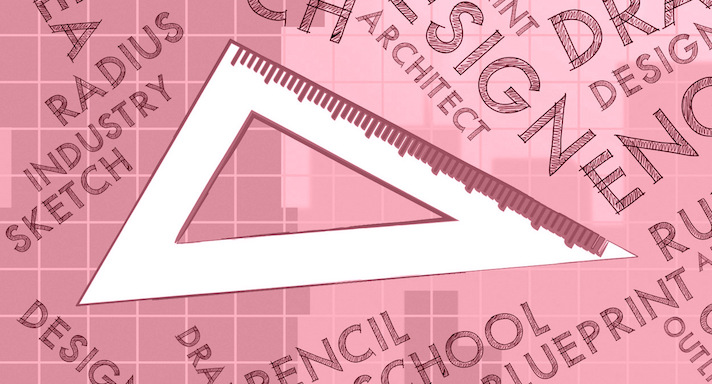High School Math III-SECourse Overview

Acellus High School Math III-SE provides Special Ed students with a basic mathematical foundation. Among the many concepts covered in this course include practice with addition and subtraction and understanding place value. Students learn how to round and are introduced to multiplication, division, and fractions. Acellus High School Math III-SE is taught by Acellus Instructor Tricia Madden.This course was developed by the International Academy of Science. Learn More

Scope and Sequence

Unit 1 In this unit students review addition, subtraction, multiplication, and division. They discuss place value up to billions and down to thousandths, as well as comparing and ordering decimals, and decimals in expanded form. Unit 2 In this unit students study mental math, the commutative and associative properties, rounding of whole numbers and decimals, and estimation of whole number and decimal sums and differences. They also explore multi-step story problems. Unit 3 In this unit students focus on multiplication. They study its properties, as well as mental math, and estimating products. They practice multiplying one- and two-digit numbers, and larger numbers. Unit 4 In this unit students focus on division. They practice dividing tens and hundreds, and discuss estimation. They consider reasonableness and quotients containing zeros. Unit 5 In this unit students continue to explore division. They study division strategies, estimating skills, quotients of different sizes, and math in real-world applications. Unit 6 In this unit students are introduced to concepts of algebra. They discuss variables, expressions, and order of operations. They study expressions with brackets, and numerical patterns. Unit 7 In this unit students focus on mutiplying decimals. They explore estimating products and multiplying decimals by powers of ten, by whole numbers, and by decimals. After this unit students are presented with the Mid-term Review and Final. Unit 8 In this unit students' emphasis is on dividing decimals. In regard to this, they explore powers of ten, exponents, estimating quotients, and dividing decimals by whole numbers. They investigate decimal divisors and dividends, and real-world, multi-step problems. Unit 9 In this unit students explore geometry. They learn about measuring and classifying. They study angles, triangles, quadrilaterals, squares, and rectangles. Unit 10 In this unit students focus on fractions. They study equivalent fractions, division with fractions, mixed numbers, simplest form, and changing fractions to decimals. Unit 11 Continuing their study of fractions, in this unit students explore addition and subtraction, like denominators, least common multiples, and least common denominators. They also discuss using benchmark fractions for estimation. Unit 12 In this unit students focus on mixed numbers. They study estimating, addition, and subtraction of mixed numbers. Unit 13 In this unit students investigate multiplication and division involving fractions. They explore multiplication and division with whole numbers, estimation, finding area of rectangles involving fractions, and multiplying mixed numbers. Unit 14 Expanding their knowledge of geometry, in this unit students explore solid figures and volume. They learn to model volume of regular and irregular objects. Unit 15 In this unit students focus on length, capacity, weight, and mass. They study customary and metric units, and practice converting each of these, both in simple and in multi-step problems. Unit 16 In this unit students explore statistics. They discuss surveys and data collection, line plots, solving problems, and ordered pairs. They learn about graphs, interpreting patterns, and comparing numerical patterns. Following this unit, students are presented with the final review and exam.